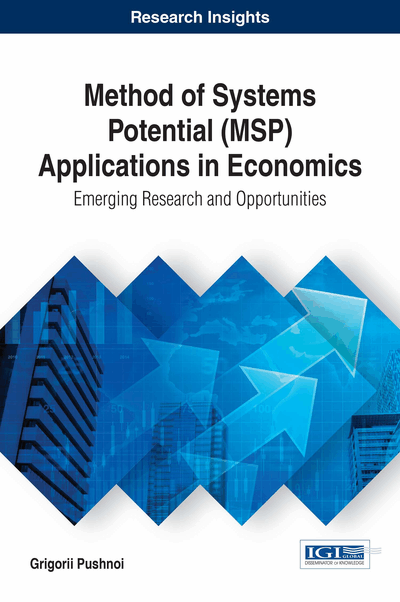# MSP-Systems with Variable Parameters

DOI: 10.4018/978-1-5225-2170-9.ch004
Available
\$37.50
No Current Special Offers

Top

## Msp-Equations And Principle Of Maximization

In this chapter we consider generalization of Method of Systems Potential for the case of systems with variable evolutionary parameters. At first we consider general mathematical formulation of this task. In MSP-model of economic CAS evolutionary parameters of economic system, economic parameters, change with time. Rate of growth,, is very unstable value. The norm of investment (investment as a percentage of GDP)and depreciation rateare much more stable values.

Although variation of parameters obviously depends partially from random factors we think that this is not a unique cause. By using stylized facts for the U.S. economy we shall show below that correlation between variations of different parameters exists in economy. Therefore the model with constant evolutionary parameters is only approximate, rough model of reality.

MSP-equations were deduced from Lamarck’s evolutionary laws. We didn’t use assumption about constancy of evolutionary parameters when we deduced MSP-equations (E1)-(E7) (Introduction). Assuming constancy of evolutionary parameters we obtained analytical solutions (13)-(21) of the equations (E1)-(E7) (Introduction).

Thus MSP-equations (E1)-(E7) are fulfilled even if evolutionary parameters are not constants. We interpret these equations as mathematical expressions of fundamental laws of evolution. These are laws which regulate the development of complex adaptive systems at macroscopic level. As a rule, fundamental laws in science express the action of the principle of maximization or of optimization of some criteria. For example laws of motion in physics follows from the principle of least action. Many of equations in economic modelling follows from the principle of maximization (of utility, profit, welfare and so on) or from the principle of minimization (of cost, expenditures and so on). Therefore it will be natural to assume that MSP-equations also can be deduced from the principle of maximization or minimization. Let’s assume that MSP-equations follow from the principle of maximization of a goal-function. We don’t discuss what sense this function makes until the application of MSP-model with variable parameters in economics will be considered.

Let’ designate goal-function as. We consider only a system of independent MSP-equations (E1), (E3) and (E4) (Introduction) for variables. The equation (E2) follows from the equations (E1) and (E3). The equation (E5) follows from the equations (E1) and (E3). The equation (E6) follows from the equations (E3) and (E4). The equation (E7) follows from the equations (E5) and (E6). Graphically a goal-functionis some kind of surface in 4-dimensional space. Vector of gradient,in definite point of 3-dimensional spacepoints in the direction of the greatest rate of increase of the goal-function. Vectordescribes the shift of location of the system in spacein unit of time. If we fix the length of this vector then vector of gradient for the goal-function determines the direction of the most rapid increase of this function. Let’s assume that MSP-equations describe motion of the system along the vector of gradientof the goal-function. It means that vectorsandare collinear. Moreover we can always normalize the goal-function in such a way that these vectors will be equal.

## Complete Chapter List

Search this Book:
Reset